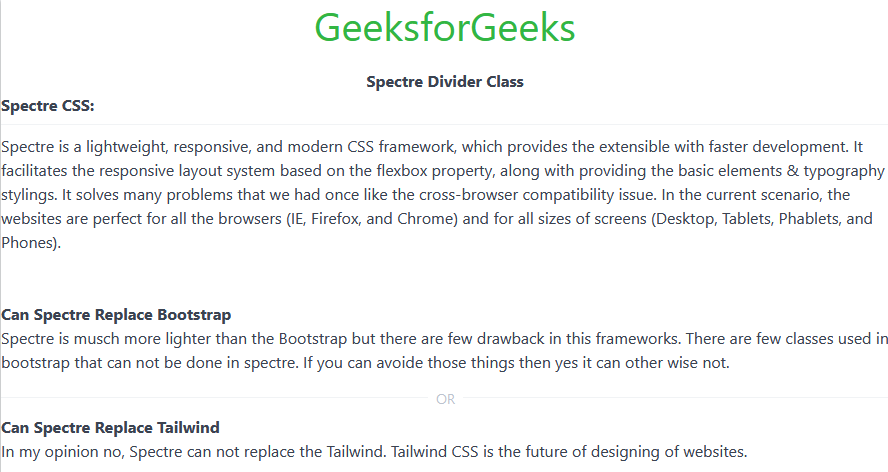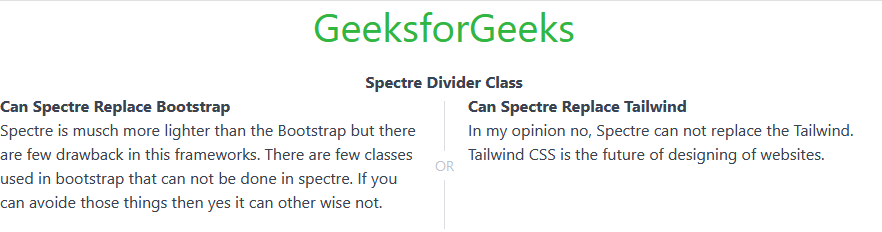GFG App
Open AppBrowser
Continue

The Spectre Divider is used to create a divider between similar kinds of components if you design login and Signup on the page but want to divide them side by side vertically or horizontally, then we can do that.

Spectre Divider Class:

• divider: This class is used to divide the contents.
• divider-vert: This class is used to create a vertical divider between contents.

Spectre Divider attribute:

• data-content: This attribute holds the text value you want to show on the divider line.

Syntax:

```<div class="divider">
....
</div>```
•  Element with text:
```<div class="divider text-center" data-content="OR">
....
</div>```

The below examples illustrate the Spectre Divider.

Example 1: In this article, we will see without text and with the text divider.

## HTML

 `` `<``html``>`   `<``head``>` `    ``<``link` `rel``=``"stylesheet"` `href``=` `"https://unpkg.com/spectre.css/dist/spectre.min.css"``>` `    ``<``link` `rel``=``"stylesheet"` `href``=` `"https://unpkg.com/spectre.css/dist/spectre-exp.min.css"``>` `    ``<``link` `rel``=``"stylesheet"` `href``=` `"https://unpkg.com/spectre.css/dist/spectre-icons.min.css"``>` ``   `<``body``>` `    ``<``center``>` `        ``<``h1` `class``=``"text-success"``>GeeksforGeeks` `        ``<``strong``>Spectre Divider Class` `        ``<``br``>` `    ``` `    ``<``strong``>Spectre CSS:` `    ``<``div` `class``=``"divider"``>` `    `  `<``p``>` `        ``Spectre is a lightweight, responsive, ` `        ``and modern CSS framework, which ` `        ``provides the extensible with faster ` `        ``development. It facilitates the ` `        ``responsive layout system based on ` `        ``the flexbox property, along with` `        ``providing the basic elements & ` `        ``typography stylings. It solves many ` `        ``problems that we had once like the ` `        ``cross-browser compatibility issue. ` `        ``In the current scenario, the ` `        ``websites are perfect for all the ` `        ``browsers (IE, Firefox, and Chrome) ` `        ``and for all sizes of screens ` `        ``(Desktop, Tablets, Phablets, and Phones).` `    ```     `    ``<``br``>` `    ``<``strong``>Can Spectre Replace Bootstrap` `    `  `<``p``>` `        ``Spectre is much more lighter than the ` `        ``Bootstrap but there are few drawback in` `        ``this frameworks. There are few classes ` `        ``used in bootstrap that can not be done ` `        ``in spectre. If you can avoid those ` `        ``things then yes it can other wise not.` `    ```     `    ``` `    ``<``div` `class``=``"divider text-center"` `data-content``=``"OR"``>` `    ``<``strong``>Can Spectre Replace Tailwind` `    `  `<``p``>` `        ``In my opinion, no, Spectre can not ` `        ``replace the Tailwind. Tailwind CSS ` `        ``is the future of designing of websites.` `    ```   ``   ``

Output:Example 2: In this example, we will see the divider-vert class.

## HTML

 `` `<``html``>`   `<``head``>` `    ``<``link` `rel``=``"stylesheet"` `href``=` `"https://unpkg.com/spectre.css/dist/spectre.min.css"``>` `    ``<``link` `rel``=``"stylesheet"` `href``=` `"https://unpkg.com/spectre.css/dist/spectre-exp.min.css"``>` `    ``<``link` `rel``=``"stylesheet"` `href``=` `"https://unpkg.com/spectre.css/dist/spectre-icons.min.css"``>` ``   `<``body``>` `    ``<``center``>` `        ``<``h1` `class``=``"text-success"``>GeeksforGeeks` `        ``<``strong``>Spectre Divider Class` `        ``<``br``>` `    ``    ` `    ``` `    ``<``div` `class``=``"columns"``>` `      ``<``div` `class``=``"column"``>` `        ``<``strong``>Can Spectre Replace Bootstrap` `           `  `<``p``>` `               ``Spectre is much more lighter than the Bootstrap ` `               ``but there are few drawback in this frameworks.` `               ``There are few classes used in bootstrap that ` `               ``can not be done in spectre. If you can avoid those ` `               ``things then yes it can other wise not.` `           ```   `      ``` `      `  `      ``<``div` `class``=``"divider-vert"` `data-content``=``"OR"``>` `      ``` `      ``<``div` `class``=``"column"``>` `          ``<``strong``>Can Spectre Replace Tailwind` `        `  `<``p``>` `            ``In my opinion no, Spectre can not replace ` `            ``the Tailwind. Tailwind CSS is the future ` `            ``of designing of websites.` `        ```   `      ``` `    ``` `` ``

Output:My Personal Notes arrow_drop_up